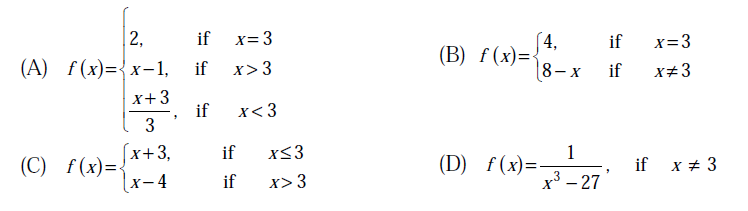# GATE | GATE CS 2013 | Question 22

• Difficulty Level : Easy
• Last Updated : 28 Jun, 2021

Which one of the following functions is continuous at x = 3?(A) A
(B) B
(C) C
(D) D

Explanation: A function is continuous at some point c,

Value of f(x) defined for x > c = Value of f(x) defined for x < c = Value of f(x) defined for x = c

All values are 2 in option A

Quiz of this Question

My Personal Notes arrow_drop_up
Recommended Articles
Page :